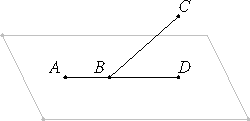# Proposition 1

A part of a straight line cannot be in the plane of reference and a part in plane more elevated.

For, if possible, let a part AB of the straight line ABC be in the plane of reference, and a part BC be in a plane more elevated.Then there is in the plane of reference some straight line continuous with AB in a straight line. Let it be BD. Therefore AB is a common segment of the two straight lines ABC and ABD, which is impossible, since, if we describe a circle with center B and radius AB, then the diameters cut off unequal circumferences of the circle.

Therefore, a part of a straight line cannot be in the plane of reference and a part in plane more elevated.

Q.E.D.

## Guide

Not only is the proof of this proposition unclear, so is the statement of it. The meaning of the “plane of reference” and the role it is to play in solid geometry are unclear. Is the intent of the statement that if part of a line lies in a plane, then all of it does? At least that would be a meaningful statement.

The proof of this proposition is unclear for more than one reason. Before a circle with center B and radius AB can be described, a plane has to be specified in which to describe the circle. In space there are infinitely many circles that have the same center B, the same radius AB, and even contain the point A. Indeed, this possibility of many circles with with same diameter was used to define a sphere in definition XI.Def.14. The last statement about unequal circumferences is incomprehensible.

The problem is that there are no postulates for solid geometry. The postulates in Book I apparently refer to an ambient plane. Certainly Post.3, “to describe a circle with any center and radius,” and Post.5 (which refers to interior angles when one line crosses two others) do. Without any postulates for nonplanar geometry it is impossible for solid geometry to get off the ground.

#### Use of this proposition

This proposition is used in the proof of the next one as well as others in the last three books of the Elements.